Like   Tweet   Pin   +1

# Happy May - New Kits and Tutorials

 table div table+table+table div table{width:100%;padding:0}table div table+table+table div table table{padding:0;float:left!important;width:51.886%!important}table div table+table+table div table table+table td,table div table+table+table div table td{padding-left:0;padding-right:0}table div table+table+table div table table td{padding-left:0;padding-right:20px}table div table+table+table div table table+table{float:left!important;width:48.114%!important}/* styles */
 table div table+table+table+table div table{width:100%;padding:0}table div table+table+table+table div table table{padding:0;float:left!important;width:51.886%!important}table div table+table+table+table div table table+table td,table div table+table+table+table div table td{padding-left:0;padding-right:0}table div table+table+table+table div table table td{padding-left:0;padding-right:20px}table div table+table+table+table div table table+table{float:left!important;width:48.114%!important}/* styles */

# Kumihimo Bracelet & Tassel with Gemstone Chip Beads

Make a bracelet and a tassel with the leftover beads. Two gemstone chip bead selections are currently offered: Larimar and Amethyst. Larimar is a gemstone found only in the Dominican Republic that has a sky blue turquoise color reminiscent of a day on the beach. Amethyst was added in honor of Ultra Violet the Pantone Color of the Year.

The bracelet has a dual side, with chips just on the top side and softer round 8s against the skin for softness. The bracelet also features a button and loop closure, but the way it is done is not the usual way starting at the button and finishing at the loop. Get the kit and find out how it saves on hand stitching. More info > Larimar & Amethyst Bracelet and Tassel Kit and Tutorial

This new kit was inspired by strands of Larimar I bought at the very last BABE show, the Bay Area Bead Extravaganza last November. After I made some of the samples, I realized the tassel could easily be turned into beautiful neckpiece with the addition a 3-ply cord and it led to a full update of the Cord Making Tutorial and Tool Set. Check Cord Making listed below. - Marion

 table div table+table+table+table+table+table div table{width:100%;padding:0}table div table+table+table+table+table+table div table img{width:96.23%;padding:0;float:none}table div table+table+table+table+table+table div table td{width:100%;padding:0 1.88% 18px}/* styles */# Cord Making - Tutorial and Tool Set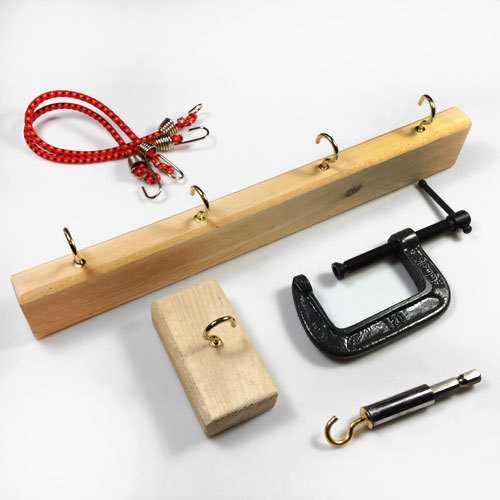/* styles */ 2-ply, 3-ply and 3-ply cord making just got a major updates. The tutorial now includes 3 projects and some brand new techniques well worth learning about. > Cord Making Tutorial & Tool Set + 3 Projects At some point I discovered that I would never be able to buy ready made cords that would match with the jewelry I was making with bonded nylon and silk, so I decided that to learn how to make my own cords. I taught myself how to make 3-ply cords and hand braided cords. I am still learning techniques along the way and just had a real breakthrough with the cable bracelets, and I feel I have just scratched the surface as to possibilities! - Marion

# Project 1 - Adjustable 3-Ply Twisted Cords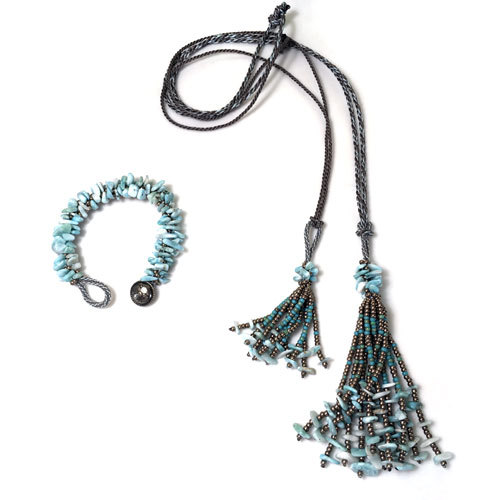...or an adjustable cord for a tassel pendant.

# Project 2 - 3-Ply Cord with Clasps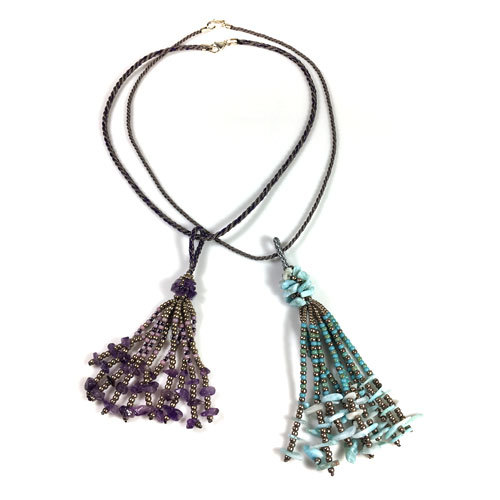A shorter cord with a claps for the tassel...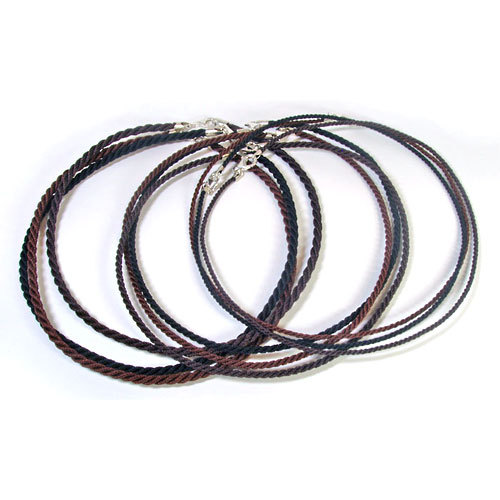... or many cords with clasp for pendants.

# Project 3 - Cable Bracelets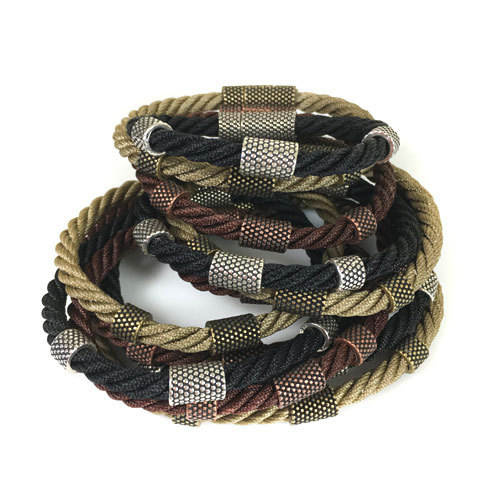Single Cable Bracelets with Dot Magnet Clasps and Sliders...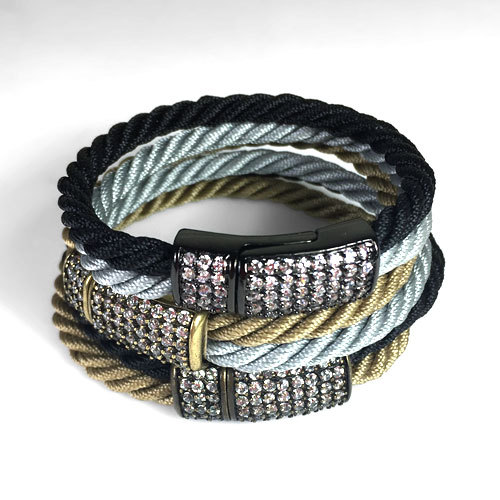Or Double Cable Bracelets with Crystal Magnet Clasps.
 /* styles */ Learn how to make your own 2-ply, 3-ply, and 4-ply twisted cord, plus get inspired by the 3 DIY projects included with step by step instructions. The full manual is 34 page long and has 67 photos, tables and charts. The Gemstone Chip Bead Bracelet & Tassel Kit includes 2 full spools of C-Lon Bead Cord, so enough cord to experiment with handmade cording. > Cord Making & DIY Projects
 table div table+table+table+table+table+table+table+table+table+table+table+table+table+table+table+table+table div table{width:100%;padding:0}table div table+table+table+table+table+table+table+table+table+table+table+table+table+table+table+table+table div table img{width:96.23%;padding:0;float:none}table div table+table+table+table+table+table+table+table+table+table+table+table+table+table+table+table+table div table td{width:100%;padding:0 1.88% 18px}/* styles */# New Solid Colors and Variegated 100% Nylon Ribbon

 table div table+table+table+table+table+table+table+table+table+table+table+table+table+table+table+table+table+table+table div table,table.module-18{width:35.28%;float:right;padding:0}table div table+table+table+table+table+table+table+table+table+table+table+table+table+table+table+table+table+table+table div table a{border:0 none;text-decoration:none}table div table+table+table+table+table+table+table+table+table+table+table+table+table+table+table+table+table+table+table div table img{width:100%!important;border:0 none;text-decoration:none}table div table+table+table+table+table+table+table+table+table+table+table+table+table+table+table+table+table+table+table div table td{width:100%;padding:0 0 20px 20px}/* styles */ All the new colors have been added + extra ones available in limited quantities. Check out the selections while they last. > 46 solid and variegated colors are available!
 table div table+table+table+table+table+table+table+table+table+table+table+table+table+table+table+table+table+table+table+table div table{width:100%;padding:0}table div table+table+table+table+table+table+table+table+table+table+table+table+table+table+table+table+table+table+table+table div table img{width:96.23%;padding:0;float:none}table div table+table+table+table+table+table+table+table+table+table+table+table+table+table+table+table+table+table+table+table div table td{width:100%;padding:0 1.88% 18px}/* styles */# No longer interested in this newsletter? Unsubscribe!

 table div table+table+table+table+table+table+table+table+table+table+table+table+table+table+table+table+table+table+table+table+table+table div table{width:100%;padding:0}table div table+table+table+table+table+table+table+table+table+table+table+table+table+table+table+table+table+table+table+table+table+table div table img{width:96.23%;padding:0;float:none}table div table+table+table+table+table+table+table+table+table+table+table+table+table+table+table+table+table+table+table+table+table+table div table td{width:100%;padding:0 1.88% 18px}/* styles */table div table+table+table+table+table+table+table+table+table+table+table+table+table+table+table+table+table+table+table+table+table+table+table+table div table{width:100%;padding:0}table div table+table+table+table+table+table+table+table+table+table+table+table+table+table+table+table+table+table+table+table+table+table+table+table div table img{width:96.23%;padding:0;float:none}table div table+table+table+table+table+table+table+table+table+table+table+table+table+table+table+table+table+table+table+table+table+table+table+table div table td{width:100%;padding:0 1.88% 18px}/* styles */